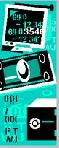Financial Terms Sensitivity

Definition of SensitivitySensitivity

The "what if" relationship between variables; the degree to which
changes in one variable cause changes in another variable. A specific synonym
is volatility.

Related Terms:

Sensitivity analysis

Analysis of the effect on a project's profitability due to changes in sales, cost, and so on.

Sensitivity analysis

An approach to understanding how changes in one variable of cost–volume–profit analysis are affected by changes in the other variables.

sensitivity analysis

a process of determining the amount of change that must occur in a variable before a different decision would be made

sensitivity analysis

Analysis of the effects of changes in sales, costs, and so on, on project profitability.

portfolio.

Duration

A common gauge of the price sensitivity of an asset or portfolio to a change in interest rates.

Greeks

Collectively, "greeks" refer to the financial measures delta, gamma,
lambda, rho, theta, and vega, which are sensitivity measures used in
evaluating derivatives.Macaulay duration

A widely used measure of price sensitivity to yield
changes developed by Frederick Macaulay in 1938. It is measured in years and
is a weighted average-time-to-maturity of an instrument. The Macaulay
duration of an income stream, such as a coupon bond, measures how long, on
average, the owner waits before receiving a payment. It is the weighted
average of the times payments are made, with the weights at time T equal to
the present value of the money received at time T.

Volatility

a. Another general term for sensitivity. b. The standard deviation
of the annualized continuously compounded rate of return of an asset. c. A
measure of uncertainty or risk.